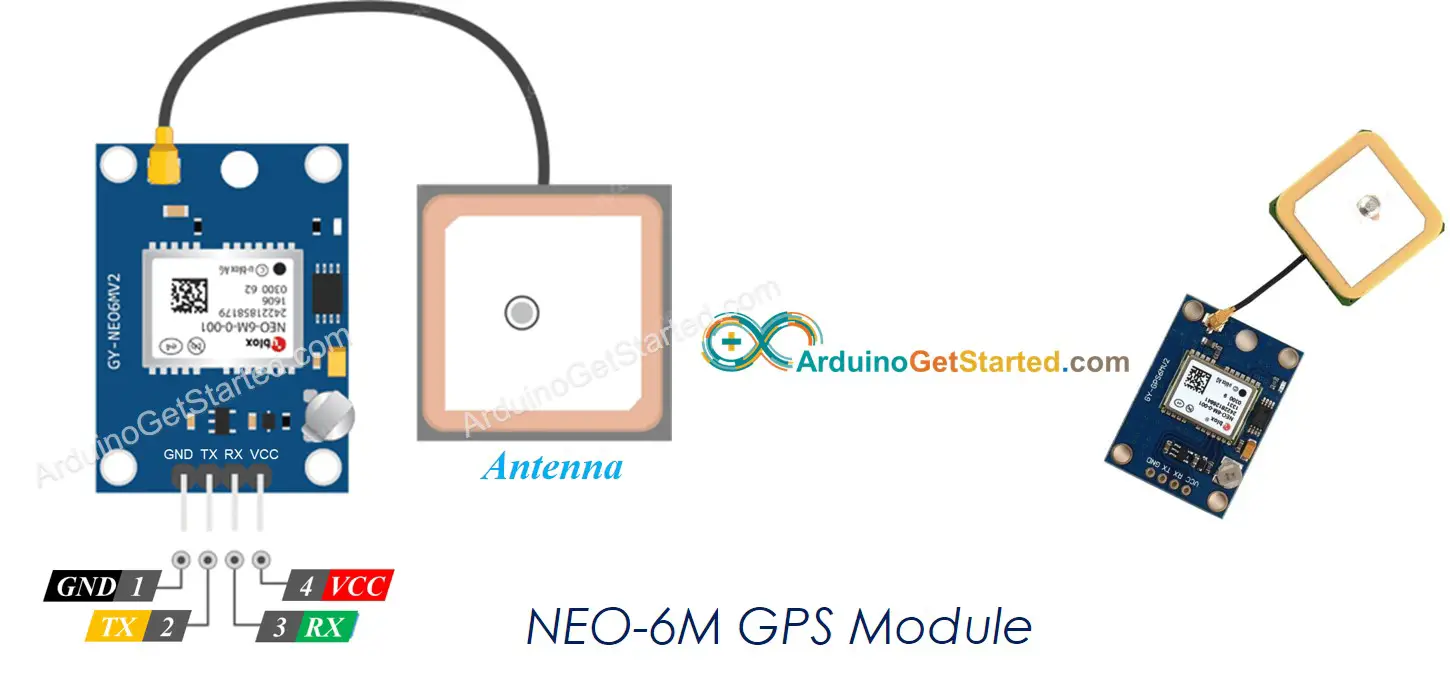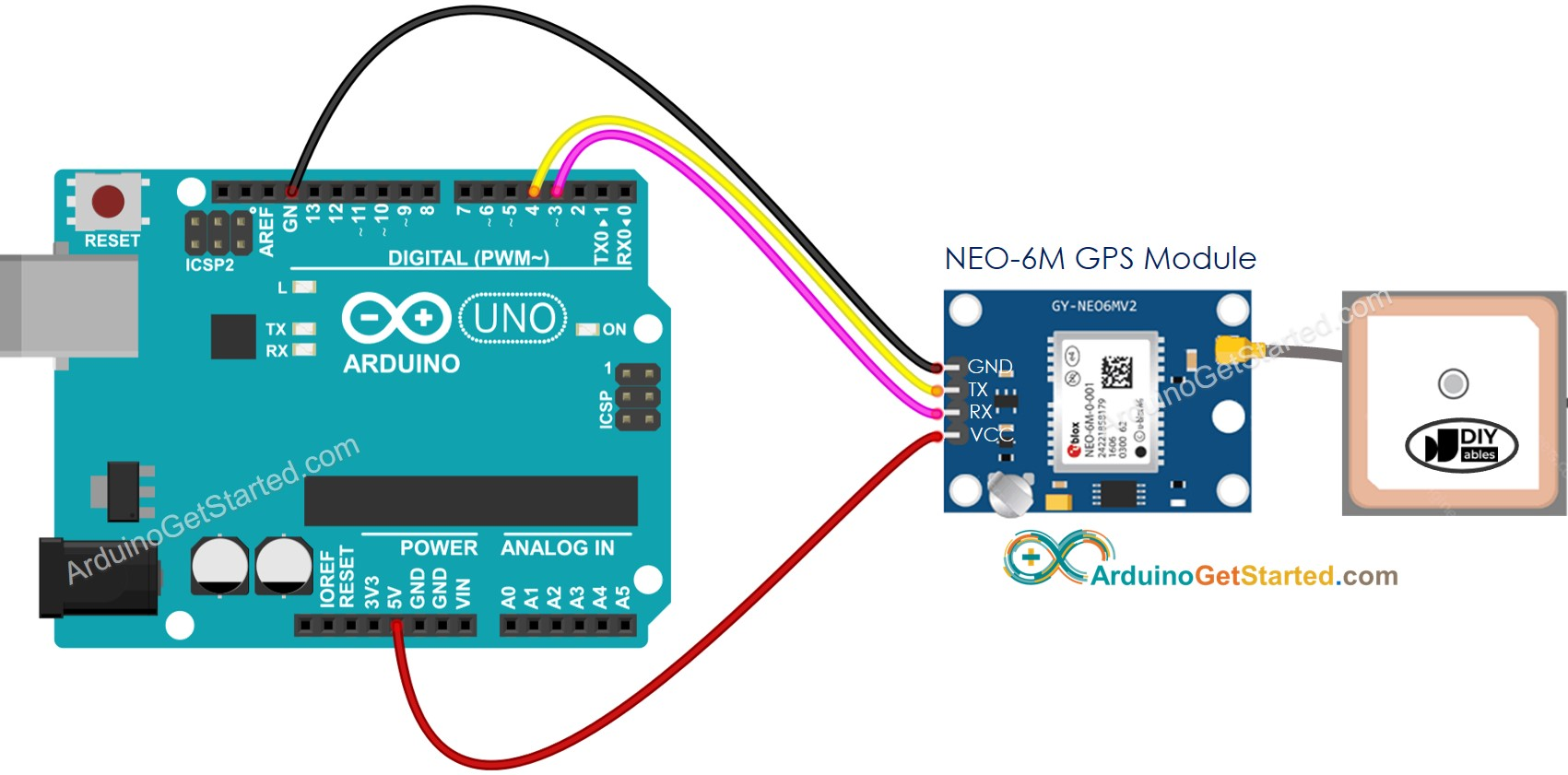# Arduino - GPS

In this tutorial, we are going to learn how to get GPS coordinates (longitude, latitude, altitude), GPS speed (km/h), and date time from NEO-6M GPS module. We also learn how to calculate the distance from current GPS position to a predefined GPS coordinates (ex. coordinates of London)

## Hardware Required

Or you can buy the following sensor kit:

Please note: These are affiliate links. If you buy the components through these links, We may get a commission at no extra cost to you. We appreciate it.

### Pinout

The NEO-6M GPS module includes 4 pins:

• VCC pin: needs to be connected to VCC (5V)
• GND pin: needs to be connected to GND (0V)
• TX pin: is used for serial communication, needs to be connect to Serial (or SoftwareSerial) RX pin on Arduino.
• RX pin: is used for serial communication, needs to be connect to Serial (or SoftwareSerial) TX pin on Arduino.## Wiring DiagramThis image is created using Fritzing. Click to enlarge image

## Arduino Code

### Reading GPS coordinates, speed (km/h), and date time

/* * Created by ArduinoGetStarted.com * * This example code is in the public domain * * Tutorial page: https://arduinogetstarted.com/tutorials/arduino-gps */ #include <TinyGPS++.h> #include <SoftwareSerial.h> const int RXPin = 3, TXPin = 4; const uint32_t GPSBaud = 9600; //Default baud of NEO-6M is 9600 TinyGPSPlus gps; // the TinyGPS++ object SoftwareSerial gpsSerial(RXPin, TXPin); // the serial interface to the GPS device void setup() { Serial.begin(9600); gpsSerial.begin(GPSBaud); Serial.println(F("Arduino - GPS module")); } void loop() { if (gpsSerial.available() > 0) { if (gps.encode(gpsSerial.read())) { if (gps.location.isValid()) { Serial.print(F("- latitude: ")); Serial.println(gps.location.lat()); Serial.print(F("- longitude: ")); Serial.println(gps.location.lng()); Serial.print(F("- altitude: ")); if (gps.altitude.isValid()) Serial.println(gps.altitude.meters()); else Serial.println(F("INVALID")); } else { Serial.println(F("- location: INVALID")); } Serial.print(F("- speed: ")); if (gps.speed.isValid()) { Serial.print(gps.speed.kmph()); Serial.println(F(" km/h")); } else { Serial.println(F("INVALID")); } Serial.print(F("- GPS date&time: ")); if (gps.date.isValid() && gps.time.isValid()) { Serial.print(gps.date.year()); Serial.print(F("-")); Serial.print(gps.date.month()); Serial.print(F("-")); Serial.print(gps.date.day()); Serial.print(F(" ")); Serial.print(gps.time.hour()); Serial.print(F(":")); Serial.print(gps.time.minute()); Serial.print(F(":")); Serial.println(gps.time.second()); } else { Serial.println(F("INVALID")); } Serial.println(); } } if (millis() > 5000 && gps.charsProcessed() < 10) Serial.println(F("No GPS data received: check wiring")); }

#### Quick Steps

• Open Arduino IDE
• On Arduino IDE, Go to Sketch Include Library Add .ZIP Library...• Select the downloaded zip file, and then the library will be installed
• Copy the above code and open with Arduino IDE
• Click Upload button on Arduino IDE to upload code to Arduino
• See the result on Serial Monitor:
COM6
Autoscroll Show timestamp
Clear output
9600 baud
Newline

### Calculating the distance from current location to a predefined location

The below code calculates the distance between the current location and London (lat:51.508131 , long: -0.128002)

/* * Created by ArduinoGetStarted.com * * This example code is in the public domain * * Tutorial page: https://arduinogetstarted.com/tutorials/arduino-gps */ #include <TinyGPS++.h> #include <SoftwareSerial.h> const int RXPin = 3, TXPin = 4; const uint32_t GPSBaud = 9600; //Default baud of NEO-6M is 9600 TinyGPSPlus gps; // the TinyGPS++ object SoftwareSerial gpsSerial(RXPin, TXPin); // the serial interface to the GPS device const double LONDON_LAT = 51.508131; const double LONDON_LON = -0.128002; void setup() { Serial.begin(9600); gpsSerial.begin(GPSBaud); Serial.println(F("Arduino - GPS module")); } void loop() { if (gpsSerial.available() > 0) { if (gps.encode(gpsSerial.read())) { if (gps.location.isValid()) { double latitude = gps.location.lat(); double longitude = gps.location.lng(); unsigned long distanceKm = TinyGPSPlus::distanceBetween(latitude, longitude, LONDON_LAT, LONDON_LON) / 1000; Serial.print(F("- latitude: ")); Serial.println(latitude); Serial.print(F("- longitude: ")); Serial.println(longitude); Serial.print(F("- distance to London: ")); Serial.println(distanceKm); } else { Serial.println(F("- location: INVALID")); } Serial.println(); } } if (millis() > 5000 && gps.charsProcessed() < 10) Serial.println(F("No GPS data received: check wiring")); }

#### Quick Steps

• Copy the above code and open with Arduino IDE
• Click Upload button on Arduino IDE to upload code to Arduino
• See the result on Serial Monitor:
COM6
Autoscroll Show timestamp
Clear output
9600 baud
Newline

## Video Tutorial

We are considering to make the video tutorials. If you think the video tutorials are essential, please subscribe to our YouTube channel to give us motivation for making the videos.

WARNING

Note that this tutorial is incomplete. We will post on our Facebook Page when the tutorial is complete. Like it to get updated.